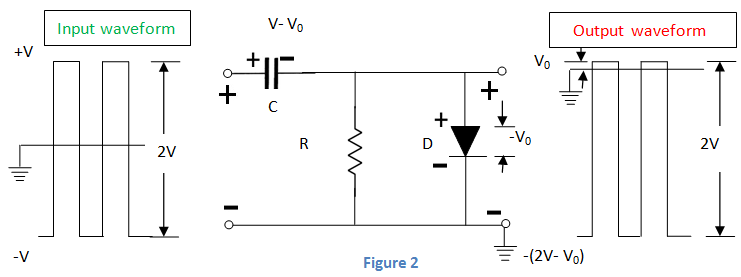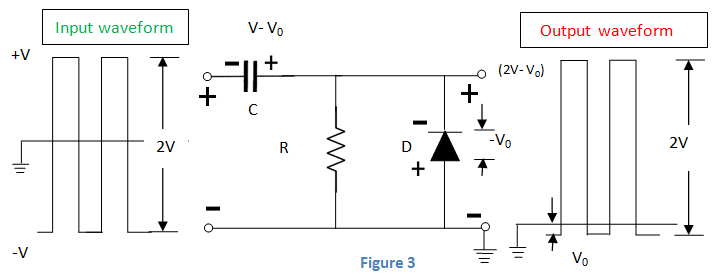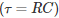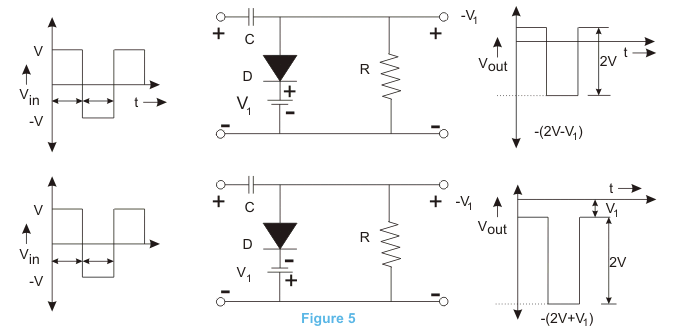# Clamper Circuit: What is it? (Diode & Voltage Clamping Circuit)

Contents

## What is a Clamper Circuit?

A clamper circuit (also known as a clamping circuit or clamper) is an electronic circuit that fixes either the positive or the negative peak values of a signal to a defined value by shifting the signal’s DC value. Clamper circuits do not restrict the peak-to-peak values of the signal. Instead, it moves the entire signal up or down to place the peaks at the desired reference level.

Clamper circuits can also be referred to as Baseline Stabilizers, DC Reinserters, Level Shifter, or D.C Restorers (these names describe the function of the circuit a bit more clearly than just “clamper”).

Clamper circuits will add the DC element, positive or negative, to the AC input signal. It pushes the signal towards the positive or the negative side (as shown in the figures below).Here, when it shifts the signal to the upward or positive sides, both the negative peak and the zero levels will meet, which is called the positive clamper circuit (top part of the above figure).

When it shifts the signal to the downside or negative side, both the positive peak and the zero levels will meet, which we refer to as the negative clamper circuit (bottom part of the above figure).

The minimum number of components of a clamping circuit is three—a capacitor, a resistor, and a diode. In some cases, a DC supply is also needed to give an additional shift.

The nature of the waveform remains the same, but the difference is in the shifted level (up or down). The peak to the peak value of the waveform will never change.

The peak value and average value of the input waveform and the clamped output will be different. The time constant of the circuit (RC) must have to be ten times the time period of the entering (input) AC voltage for the better clamping action.

Let’s look at a diode negative clamper, shown in figure 2 below.Throughout the positive half cycle of input, the D diode will conduct, and the output voltage will be the same as the barrier potential of the diode (V0).

At that time, the capacitor will get charged to (V – V0). Throughout the negative half cycle of input, the diode will become negative biased, and it has no role on capacitor voltage. The capacitor cannot discharge a lot because of the high value of R.

Therefore, the output voltage will be – (2V- V0). The peak-to-peak voltage will be 2V. The output waveform that we get will be the original signal shifted in the downward direction.

Next, let’s look at a positive diode clamper shown in figure 3 below. The only distinction from the previous circuit is that diode is in reverse polarity. So the output will be the shifted original signal in the upward direction.

Its underlying function is the same as the negative clamper circuit above the clamper circuit.

This time though, the diode is pointing in the upward direction, not downwards. This means that the current is now flowing in the opposite direction.

Thus we can conclude that we obtain the positive clamper if the diode in the circuit points in the upward direction, and we get a negative camper when the diode points in the downward direction.## How Does a Clamping Circuit Work?

In general, the clamper circuit depends on a variation in the capacitor time constant.The time constant should be sufficient that the capacitor voltage does not discharge considerably throughout the non-conducting diode period.

One should select the values of capacitance and resistance so that the circuit keeps the time constant high. For preventing quick discharge of the capacitor, the resistance value should be high.

All through the diode conducting period, the capacitor charging should be high-speed. For this, we select a small value of capacitance.

The C in the positive clamper charges quickly throughout the first negative phase of AC input voltage. When Vin becomes +ve, the C serves as a voltage doubler, and when Vin is –ve, the C operates as a battery with voltage Vin.

Thus, we can conclude that the capacitor and input voltage act against each other. This results in zero net voltage as seen by the load.

The biased positive clamping circuit and biased negative clamping circuit are shown in Figures 4 and 5.Want To Learn Faster? 🎓
Get electrical articles delivered to your inbox every week.
No credit card required—it’s 100% free.